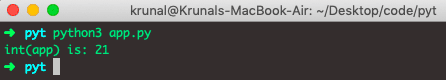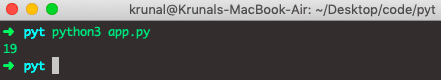# Python int: The Complete Guide

The int() function in Python and Python3 converts the number in the given base to decimal. The int() function converts the specified value into an integer number.

## Python int

Python int() is a built-in method that returns an integer object from any number or string. The int() method accepts value and base as arguments and returns an integer value.

### Syntax

`int(value, base)`

### Parameters

value parameter is a number or a string that can be converted into an integer number.

A base parameter is a number representing the number format. The default value is 10.

See the following example.

```# app.py

data = "1921"
print(int(data))```

See the output.So, the int() method returns:

1. The integer object from a given number or string treats the default base as 10.
2. If there are no parameters, then it returns 0
3. If the base has been given, it treats the string in the given base (0, 2, 8, 10, 16).

## Python int() for custom objects

Internally, the int() method calls an object’s __int__() method.

So, even if an object isn’t a number, you can convert an object into an integer object.

You can do this by overriding a class’s __index__() and __int__() methods to return the number.

The two methods mentioned above should return the same value as an older version of Python uses an __int__(), while the newer uses an __index__() method.

See the following code.

```# app.py

class App:
price = 21

def __index__(self):
return self.price

def __int__(self):
return self.price

app = App()
print('int(app) is:', int(app))```

See the output.## How to convert float to int in Python

To convert float to int in Python, use the int() function.

```# app.py

amp = 19.21
print(int(amp))```

See the output.You could use the round function. If you use no second parameter (# of significant digits), I think you will get the behavior you want.

That’s it for this tutorial.

## Related posts

Python string to int

Python checks if a string is an integer

This site uses Akismet to reduce spam. Learn how your comment data is processed.